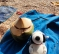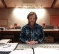## How do I produce a covariance matrix from IQ samples?Started by 11 months ago8 replieslatest reply 4 months ago307 views

Dear Colleagues,

I'm working with BLE AoA Localization. My problem is: What to do exactly with IQ(in phase-quadrature signals) information from an antenna array in order to produce a covariance matrix and then use it in MUSIC algorithm.

I've already read several articles and documentations, but they never go to the numerical details of what goes on between my IQ information and the process to build its covariance matrix. As you can see, I'm kinda new in this field. I came from Audio Signal Processing, so I'm used to build a covariance matrix from a certain number of data/samples received in each microphone from the array. In the BLE AoA case, my information is just a pair of numbers for each antenna (from which I obtain the phase and amplitude of the Sinusoid). Below, are some examples of what I'm reading right now:

https://www.digikey.com/en/articles/use-bluetooth-...

https://www.researchgate.net/publication/351812073...

btw: I'm aware of phase alignment with the reference antenna process.

Could anyone tell me if there are any specific examples that could help me out?

[ - ]If you're familiar with MATLAB, then you can work through the full example of MUSIC I originally posted here back in 2013.

The code is heavily commented and doesn't cheat by using high-level MATLAB functions. (It uses MATLAB's eig() function for computing an eigendecomposition, but that's about it). So it takes you through every step, starting from the raw complex baseband (IQ) samples.

At the link above, you can also find an example of 2D (azimuth and elevation) AoA estimation, in case that's of interest. For starters, here's the marginally simpler 1D (azimuth only) version:

close all; clear all; clc;
% ======= (1) TRANSMITTED SIGNALS ======= %

% Signal source directions
az = [35;39;127]; % Azimuths
el = zeros(size(az)); % Simple example: assume elevations zero
M = length(az); % Number of sources

% Transmitted signals
L = 200; % Number of data snapshots recorded by receiver
m = randn(M,L); % Example: normally distributed random signals

% ========= (2) RECEIVED SIGNAL ========= %

% Wavenumber vectors (in units of wavelength/2)
k = pi*[cosd(az).*cosd(el), sind(az).*cosd(el), sind(el)].';

% Array geometry [rx,ry,rz]
N = 10; % Number of antennas
r = [(-(N-1)/2:(N-1)/2).',zeros(N,2)]; % Assume uniform linear array

% Matrix of array response vectors
A = exp(-1j*r*k);

sigma2 = 0.01; % Noise variance
n = sqrt(sigma2)*(randn(N,L) + 1j*randn(N,L))/sqrt(2);

x = A*m + n;

% ========= (3) MUSIC ALGORITHM ========= %

% Sample covariance matrix
Rxx = x*x'/L;

% Eigendecompose
[E,D] = eig(Rxx);
[lambda,idx] = sort(diag(D)); % Vector of sorted eigenvalues
E = E(:,idx); % Sort eigenvalues accordingly
En = E(:,1:end-M); % Noise eigenvectors (ASSUMPTION: M IS KNOWN)

% MUSIC search directions
AzSearch = (0:1:180).'; % Azimuth values to search
ElSearch = zeros(size(AzSearch)); % Simple 1D example

% Corresponding points on array manifold to search
kSearch = pi*[cosd(AzSearch).*cosd(ElSearch), ...
sind(AzSearch).*cosd(ElSearch), sind(ElSearch)].';
ASearch = exp(-1j*r*kSearch);

% MUSIC spectrum
Z = sum(abs(ASearch'*En).^2,2);

% Plot
figure();
plot(AzSearch,10*log10(Z));
title('Simple 1D MUSIC Example');
xlabel('Azimuth (degrees)');
ylabel('MUSIC spectrum (dB)');
grid on; axis tight;
[ - ]Thank you very much for this example. Luckily I'm indeed familiar with Matlab and could understand what you've done there.

[ - ]Thanks for the insight on the implementation for matlab. I still have a doubt on how the recieved signal X is calculated based on the I/Q samples.

I understand that you need to calculate the phase difference between the antennas for Bluetooth direction finding. So based on the matlab example, the vector az would be replaced with the phase differences of all the sampling instants K, and az would have a size of 1x(K-1) ?

[ - ]Sounds beautiful. What is the purpose of the music algorithm? To compose music? Or process audio or some other processing concept?

[ - ]Unfortunately, there is no relation to music. The purpose of the Multiple Signal Classification (MUSIC) algorithm, from my perspective here, is to find the signal source direction through the Spectral Decomposition of the Covariance Matrix provided by the same signal received by each antenna in the array.

[ - ]For the covariance matrix of a complex vector, see:

https://en.wikipedia.org/wiki/Complex_random_vector

The only catch with complex numbers is taking the complex conjugate of the second term.

[ - ]Equation 2